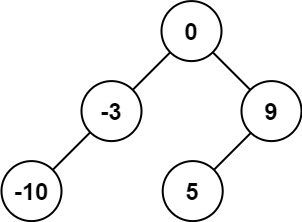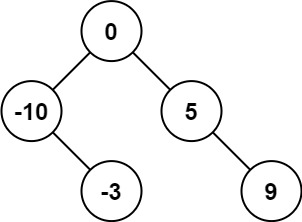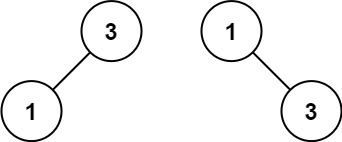# 108. Convert Sorted Array to Binary Search Tree

catepk·2021년 8월 22일
0

## LeetCode

목록 보기
50/88108. Convert Sorted Array to Binary Search Tree

### 문제

Given an integer array nums where the elements are sorted in ascending order, convert it to a height-balanced binary search tree.

A height-balanced binary tree is a binary tree in which the depth of the two subtrees of every node never differs by more than one.

Example 1:Input: nums = [-10,-3,0,5,9]
Output: [0,-3,9,-10,null,5]
Explanation: [0,-10,5,null,-3,null,9] is also accepted:Example 2:Input: nums = [1,3]
Output: [3,1]
Explanation: [1,3] and [3,1] are both a height-balanced BSTs.

Constraints:

1 <= nums.length <= 10^4
-10^4 <= nums[i] <= 10^4
nums is sorted in a strictly increasing order.

### 풀이

# Definition for a binary tree node.
# class TreeNode:
#     def __init__(self, val=0, left=None, right=None):
#         self.val = val
#         self.left = left
#         self.right = right
class Solution:
def sortedArrayToBST(self, nums: List[int]) -> Optional[TreeNode]:
if not nums:
return None
mid = len(nums) // 2
node = TreeNode(nums[mid])
node.left = self.sortedArrayToBST(nums[:mid])
node.right = self.sortedArrayToBST(nums[mid + 1:])

return node